Write your Compiler by Proving it Correct
23rd August, 2015
liamoc.net

Recently my research has been centered around the development of a self-certifying compiler for a functional language with linear types called Cogent (see O’Connor et al. (2016)). The compiler works by emitting, along with generated low-level code, a proof in Isabelle/HOL (see Nipkow et al. (2002)) that the generated code is a refinement of the original program, expressed via a simple functional semantics in HOL.

As dependent types unify for us the language of code and proof, my current endeavour has been to explore how such a compiler would look if it were implemented and verified in a dependently typed programming language instead. In this post, I implement and verify a toy compiler for a language of arithmetic expressions and variables to an idealised assembly language for a virtual stack machine, and explain some of the useful features that dependent types give us for writing verified compilers.

The Agda snippets in this post are interactive! Click on a symbol to see its definition.

## Wellformedness

One of the immediate advantages that dependent types give us is that we can encode the notion of term wellformedness in the type given to terms, rather than as a separate proposition that must be assumed by every theorem.

Even in our language of arithmetic expressions and variables, which does not have much of a static semantics, we can still ensure that each variable used in the program is bound somewhere. We will use indices instead of variable names in the style of de Bruijn (1972), and index terms by the number of available variables, a trick I first noticed in McBride (2003). The Fin type, used to represent variables, only contains natural numbers up to its index, which makes it impossible to use variables that are not available.

data Term (n : ℕ) : Set where
Lit : ℕ → Term n
_⊠_ : Term n → Term n → Term n
_⊞_ : Term n → Term n → Term n
Let_In_ : Term n → Term (suc n) → Term n
Var : Fin n → Term n


This allows us to express in the type of our big-step semantics relation that the environment E (here we used the length-indexed Vec type from the Agda standard library) should have a value for every available variable in the term. In any Isabelle specification of the same, we would have to add such length constraints as explicit assumptions, either in the semantics themselves or in theorems about them. In Agda, the dynamic semantics are extremely clean, unencumbered by irritating details of the encoding:

infixl 5 _⊢_⇓_

data _⊢_⇓_ {n : ℕ} ( E : Vec ℕ n) : Term n → ℕ → Set where
lit-e   : ∀{n}

-------------
→ E ⊢ Lit n ⇓ n

times-e : ∀{e₁ e₂}{v₁ v₂}

→ E ⊢ e₁ ⇓ v₁
→ E ⊢ e₂ ⇓ v₂
---------------------
→ E ⊢ e₁ ⊠ e₂ ⇓ v₁ * v₂

plus-e  : ∀{e₁ e₂}{v₁ v₂}

→ E ⊢ e₁ ⇓ v₁
→ E ⊢ e₂ ⇓ v₂
---------------------
→ E ⊢ e₁ ⊞ e₂ ⇓ v₁ + v₂

var-e   : ∀{n}{x}

→ E [ x ]= n
-------------
→ E ⊢ Var x ⇓ n

let-e   : ∀{e₁}{e₂}{v₁ v₂}

→ E        ⊢ e₁ ⇓ v₁
→ (v₁ ∷ E) ⊢ e₂ ⇓ v₂
---------------------
→ E ⊢ Let e₁ In e₂ ⇓ v₂


By using appropriate type indices, it is possible to extend this technique to work even for languages with elaborate static semantics. For example, linear type systems (see Walker (2005)) can be encoded by indexing terms by type contexts (in a style similar to Oleg). Therefore, the boundary between being wellformed and being well-typed is entirely arbitrary. It’s possible to use relatively simple terms and encode static semantics as a separate judgement, or to put the entire static semantics inside the term structure, or to use a mixture of both. In this simple example, our static semantics only ensure variables are in scope, so it makes sense to encode the entire static semantics in the terms themselves.

Similar tricks can be employed when encoding our target language, the stack machine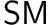. This machine consists of two stacks of numbers, the working stack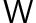and the storage stack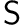, and a program to evaluate. A program is a list of instructions.

There are six instructions in total, each of which manipulate these two stacks in various ways. When encoding these instructions in Agda, we index the Inst type by the size of both stacks before and after execution of the instruction:

data Inst  : ℕ → ℕ → ℕ → ℕ → Set where
num   : ∀{w s} → ℕ → Inst w s (suc w) s
plus  : ∀{w s} → Inst (suc (suc w)) s (suc w) s
times : ∀{w s} → Inst (suc (suc w)) s (suc w) s
push  : ∀{w s} → Inst (suc w) s w (suc s)
pick  : ∀{w s} → Fin s → Inst w s (suc w) s
pop   : ∀{w s} → Inst w (suc s) w s


Then, we can define a simple type forprograms, essentially a list of instructions where the stack sizes of consecutive instructions must match. This makes it impossible to construct aprogram with an underflow error:

data SM (w s : ℕ) : ℕ → ℕ → Set where
halt : SM w s w s
_∷_  : ∀{w′ s′ w″ s″} → Inst w s w′ s′ → SM w′ s′ w″ s″ → SM w s w″ s″


We also define a simple sequential composition operator, equivalent to list append (++):

infixr 5 _⊕_
_⊕_ : ∀{w s w′ s′ w″ s″} → SM w s w′ s′ → SM w′ s′ w″ s″ → SM w s w″ s″
halt    ⊕ q = q
(x ∷ p) ⊕ q = x ∷ (p ⊕ q)


The semantics of each instruction are given by the following relation, which takes the two stacks and an instruction as input, returning the two updated stacks as output. Note the size of each stack is proscribed by the type of the instruction, just as the size of the environment was proscribed by the type of the term in the source language, which eliminates the need to add tedious wellformedness assumptions to theorems or rules.

infixl 5 _∣_∣_↦_∣_

data _∣_∣_↦_∣_ : ∀{w s w′ s′}
→ Vec ℕ w → Vec ℕ s
→ Inst w s w′ s′
→ Vec ℕ w′ → Vec ℕ s′
→ Set where


The semantics of each instruction are as follows:

•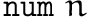(where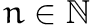), pushes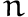to.num-e   : ∀{w s}{n}{W : Vec _ w}{S : Vec _ s}

-------------------------
→ W ∣ S ∣ num n ↦ n ∷ W ∣ S

•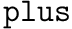, pops two numbers fromand pushes their sum back to.plus-e  : ∀{w s}{n m}{W : Vec _ w}{S : Vec _ s}

----------------------------------------
→ (n ∷ m ∷ W) ∣ S ∣ plus ↦ (m + n ∷ W) ∣ S

•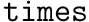, pops two numbers fromand pushes their product back to.times-e : ∀{w s}{n m}{W : Vec _ w}{S : Vec _ s}

-----------------------------------------
→ (n ∷ m ∷ W) ∣ S ∣ times ↦ (m * n ∷ W) ∣ S

•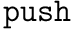, pops a number fromand pushes it to.push-e  : ∀{w s}{n}{W : Vec _ w}{S : Vec _ s}

--------------------------------
→ (n ∷ W) ∣ S ∣ push ↦ W ∣ (n ∷ S)

•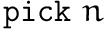(where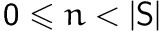), pushes the number at positionfrom the top ofonto.pick-e  : ∀{w s}{x}{n}{W : Vec _ w}{S : Vec _ s}

→ S [ x ]= n
----------------------------
→ W ∣ S ∣ pick x ↦ (n ∷ W) ∣ S

•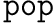, removes the top number from.pop-e   : ∀{w s}{n}{W : Vec _ w}{S : Vec _ s}

-------------------------
→ W ∣ (n ∷ S) ∣ pop ↦ W ∣ S


As programs are lists of instructions, the evaluation of programs is naturally specified as a list of evaluations of instructions:

infixl 5 _∣_∣_⇓_∣_

data _∣_∣_⇓_∣_ {w s : ℕ}(W : Vec ℕ w)(S : Vec ℕ s) : ∀{w′ s′}
→ SM w s w′ s′
→ Vec ℕ w′ → Vec ℕ s′
→ Set where

halt-e : W ∣ S ∣ halt ⇓ W ∣ S

_∷_ : ∀{w′ s′ w″ s″}{i}{is}
→ {W′ : Vec ℕ w′}{S′ : Vec ℕ s′}
→ {W″ : Vec ℕ w″}{S″ : Vec ℕ s″}

→ W ∣ S ∣ i ↦ W′ ∣ S′
→ W′ ∣ S′ ∣ is ⇓ W″ ∣ S″
--------------------------
→ W ∣ S ∣ (i ∷ is) ⇓ W″ ∣ S″



The semantics of sequential composition is predictably given by appending these lists:

infixl 4 _⟦⊕⟧_
_⟦⊕⟧_ : ∀{w w′ w″ s s′ s″}{P}{Q}
→ {W : Vec ℕ w}{S : Vec ℕ s}
→ {W′ : Vec ℕ w′}{S′ : Vec ℕ s′}
→ {W″ : Vec ℕ w″}{S″ : Vec ℕ s″}

→ W ∣ S ∣ P ⇓ W′ ∣ S′
→ W′ ∣ S′ ∣ Q ⇓ W″ ∣ S″
-------------------------
→ W ∣ S ∣ P ⊕ Q ⇓ W″ ∣ S″
halt-e ⟦⊕⟧ ys = ys
x ∷ xs ⟦⊕⟧ ys = x ∷ (xs ⟦⊕⟧ ys)


## Writing by proving

Having formally defined our source and target languages, we can now prove our compiler correct – even though we haven’t written a compiler yet!

One of the other significant advantages dependent types bring to compiler verification is the elimination of repetition. In my larger Isabelle formalisation, the proof of the compiler’s correctness largely duplicates the structure of the compiler itself, and this tight coupling means that proofs must be rewritten along with the program – a highly tedious exercise. As dependently typed languages unify the language of code and proof, we can merely provide the correctness proof: in almost all cases, the correctness proof is so specific, that the program of which it demonstrates correctness can be derived automatically.

We define a compiler’s correctness to be the commutativity of the following diagram, as per Hutton & Wright (2004).As we have not proven determinism for our semantics1, such a correctness condition must be shown by the conjunction of a soundness and completeness condition, similar to Bahr (2015).

Soundness is a proof that the compiler output is a refinement of the input, that is, every evaluation in the output is matched by the input. The output does not do anything that the input doesn’t do.

-- Sound t u means that u is a sound translation of t
Sound : ∀{w s} → Term s → SM w s (suc w) s → Set
Sound {w} t u = ∀{v}{E}{W : Vec ℕ w}

→ W ∣ E ∣ u ⇓ (v ∷ W) ∣ E
-----------------------
→ E ⊢ t ⇓ v


Note that we generalise the evaluation statements used here slightly to use arbitrary environments and stacks. This is to allow our induction to proceed smoothly.

Completeness is a proof that the compiler output is an abstraction of the input, that is, every evaluation in the input is matched by the output. The output does everything that the input does.

Complete : ∀{w s} → Term s → SM w s (suc w) s → Set
Complete {w} t u = ∀{v}{E}{W : Vec ℕ w}

→ E ⊢ t ⇓ v
-----------------------
→ W ∣ E ∣ u ⇓ (v ∷ W) ∣ E


It is this completeness condition that will allow us to automatically derive our code generator. Given a term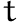, our generator will return a Σ-type, or dependent pair, containing aprogram called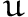and a proof thatis a complete translation of:

codegen′  : ∀{w s}
→ (t : Term s)
→ Σ[ u ∈ SM w s (suc w) s ] Complete t u


For literals, we simply push the number of the literal onto the working stack:

codegen′ (Lit x ) = _ , proof
where
proof : Complete _ _
proof lit-e = num-e ∷ halt-e


The code above never explicitly states whatprogram to produce! Instead, it merely provides the completeness proof, and the rest can be inferred by unification. Similar elision can be used for variables, which pick the correct index from the storage stack:

codegen′ (Var x) = _ , proof
where
proof : Complete _ _
proof (var-e x) = pick-e x ∷ halt-e


The two binary operations are essentially the standard translation for an infix-to-postfix tree traversal, but once again the program is not explicitly emitted, but is inferred from the completeness proof used.

codegen′ (t₁ ⊞ t₂) = _ , proof (proj₂ (codegen′ t₁)) (proj₂ (codegen′ t₂))
where
proof : ∀ {u₁}{u₂} → Complete t₁ u₁  → Complete t₂ u₂ → Complete _ _
proof p₁ p₂ (plus-e t₁ t₂) = p₁ t₁ ⟦⊕⟧ p₂ t₂ ⟦⊕⟧ plus-e ∷ halt-e

codegen′ (t₁ ⊠ t₂) = _ , proof (proj₂ (codegen′ t₁)) (proj₂ (codegen′ t₂))
where
proof : ∀ {u₁}{u₂} → Complete t₁ u₁  → Complete t₂ u₂ → Complete _ _
proof p₁ p₂ (times-e t₁ t₂) = p₁ t₁ ⟦⊕⟧ p₂ t₂ ⟦⊕⟧ times-e ∷ halt-e


The variable-binding form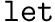pushes the variable to the storage stack and cleans up after evaluation exits the scope with.

codegen′ (Let t₁ In t₂)
= _ , proof (proj₂ (codegen′ t₁)) (proj₂ (codegen′ t₂))
where
proof : ∀ {u₁}{u₂} → Complete t₁ u₁  → Complete t₂ u₂ → Complete _ _
proof p₁ p₂ (let-e t₁ t₂)
= p₁ t₁ ⟦⊕⟧ push-e ∷ (p₂ t₂ ⟦⊕⟧ pop-e ∷ halt-e)


We can extract a more standard-looking code generator function simply by throwing away the proof that our code generator produces.

codegen : ∀{w s}
→ Term s
→ SM w s (suc w) s
codegen {w}{s} t = proj₁ (codegen′ {w}{s} t)

The soundness proof for this code generator isn’t particularly illuminating and is rather awkwardly expressed. Nevertheless, for the truly brave, you may click here to view it.

We use an alternative presentation of the soundness property, that makes explicit several equalities that are implicit in the original formulation of soundness. We prove that our new formulation still implies the original one.

open import Relation.Binary.PropositionalEquality

Sound′ : ∀{w s} → Term s → SM w s (suc w) s → Set
Sound′ {w} t u = ∀{E E′}{W : Vec ℕ w}{W′}
→ W ∣ E ∣ u ⇓ W′ ∣ E′
------------------------------------------
→ (E ≡ E′) × (tail W′ ≡ W) × E ⊢ t ⇓ head W′

sound′→sound : ∀{w s}{t}{u} → Sound′ {w}{s} t u → Sound t u
sound′→sound p x with p x
... | refl , refl , q = q


As our soundness proof requires us to do a lot of rule inversion on the evaluation ofprograms, we need an eliminator for the introduction rule _⟦⊕⟧_, used in the completeness proof, which breaks an evaluation of a sequential composition into evaluations of its component parts:

⊕-elim : ∀{w s w′ s′ w″ s″}
{W : Vec ℕ w}{S : Vec ℕ s}
{W″ : Vec ℕ w″}{S″ : Vec ℕ s″}
{a : SM w s w′ s′}{b : SM w′ s′ w″ s″}

→ W ∣ S ∣ a ⊕ b ⇓ W″ ∣ S″
→ ∃[ W′ ] ∃[ S′ ] ((W ∣ S ∣ a ⇓ W′ ∣ S′) × (W′ ∣ S′ ∣ b ⇓ W″ ∣ S″))
⊕-elim {a = halt} p = _ , _ , halt-e , p
⊕-elim {a = a ∷ as} (x ∷ p) with ⊕-elim {a = as} p
... | _ , _ , p₁ , p₂ = _ , _ , x ∷ p₁ , p₂

Then the soundness proof is given as a boatload of rule inversion and matching on equalities, to convince Agda that there is no other way to possibly evaluate the compiler output:
soundness : ∀{w s}{t : Term s} → Sound′ {w} t (codegen t)
soundness {t = Lit x} (num-e     ∷ halt-e) = refl , refl , lit-e
soundness {t = Var x} (pick-e x₁ ∷ halt-e) = refl , refl , var-e x₁
soundness {t = t₁ ⊠ t₂} x
with ⊕-elim {a = codegen t₁ ⊕ codegen t₂} x
...  | _ , _ , p , _
with ⊕-elim {a = codegen t₁} p
...  | _ , _ , p₁ , p₂
with soundness {t = t₁} p₁ | soundness {t = t₂} p₂
soundness {t = t₁ ⊠ t₂} x
| _  ∷  _ ∷  _ , ._ , _ , times-e ∷ halt-e
|      ._ ∷ ._ , ._ , _ , _
| refl , refl , a
| refl , refl , b
= refl , refl , times-e a b
soundness {t = t₁ ⊞ t₂} x
with ⊕-elim {a = codegen t₁ ⊕ codegen t₂} x
...  | _ , _ , p , _
with ⊕-elim {a = codegen t₁} p
...  | _ , _ , p₁ , p₂
with soundness {t = t₁} p₁ | soundness {t = t₂} p₂
soundness {t = t₁ ⊞ t₂} x
| _  ∷  _ ∷  _ , ._ , _ , plus-e ∷ halt-e
|      ._ ∷ ._ , ._ , _ , _
| refl , refl , a
| refl , refl , b
= refl , refl , plus-e a b
soundness {t = Let t₁ In t₂} x
with ⊕-elim {a = codegen t₁} x
...  | _ ∷ _ , _ , p₁ , push-e ∷ q
with ⊕-elim {a = codegen t₂} q
...  | _ ∷ _ , _ ∷ _ , p₂ , _
with soundness {t = t₁} p₁ | soundness {t = t₂} p₂
soundness {t = Let t₁ In t₂} x
| _ ∷ ._ , ._      , _ , push-e ∷ q
| _ ∷ ._ , ._ ∷ ._ , _ , pop-e ∷ halt-e
| refl , refl , a
| refl , refl , b
= refl , refl , let-e a b


## Compiler Frontend

Now that we have a verified code generator, as a final flourish we’ll implement a basic compiler frontend2 for our language and run it on some basic examples.

We define a surface syntax as follows. In the tradition of all the greatest languages such as BASIC, FORTRAN and COBOL, capital letters are exclusively used, and English words are favoured over symbols because it makes the language readable to non-programmers. I should also acknowledge the definite influence of PHP, Perl and sh on the choice of the $ sigil to precede variable names. The sigil # precedes numeric literals as Agda does not allow us to overload them. data Surf : Set where LET_BE_IN_ : String → Surf → Surf → Surf _PLUS_ : Surf → Surf → Surf _TIMES_ : Surf → Surf → Surf$_         : String → Surf
#_         : ℕ → Surf

infixr 4 LET_BE_IN_
infixl 5 _PLUS_
infixl 6 _TIMES_
infix 7 $_ infix 7 #_  Unlike our Term AST, this surface syntax does not include any scope information, uses strings for variable names, and is more likely to be something that would be produced from a parser. In order to compile this language, we must first translate it into our wellformed-by-construction Term type, which necessitates scope-checking. check : ∀{n} → Vec String n → Surf → Maybe (Term n) check Γ (LET x BE s IN t) = pure Let_In_ ⊛ check Γ s ⊛ check (x ∷ Γ) t check Γ (s PLUS t) = pure _⊞_ ⊛ check Γ s ⊛ check Γ t check Γ (s TIMES t) = pure _⊠_ ⊛ check Γ s ⊛ check Γ t check Γ (# x) = pure (Lit x) check Γ ($ x)             = pure Var     ⊛ find Γ x
where
find : ∀{n} → Vec String n → String → Maybe (Fin n)
find [] s = nothing
find (x ∷ v) s with s ≟ x
... | yes _ = just zero
... | no  _ = suc <$> find v s  Note that this function is the only one in our development that is partial: it can fail if an undeclared variable is used. For this reason, we use the Applicative instance for Maybe to make the error handling more convenient. Our compiler function, then, merely composes our checker with our code generator: compiler : Surf → Maybe (SM 0 0 1 0) compiler s = codegen <$> check [] s


Note that we can’t really demonstrate correctness of the scope-checking function, save that if it outputs a Termthen there are no scope errors in, as it is impossible to construct a Term with scope errors. One possibility would be to define a semantics for the surface syntax, however this would necessitate a formalisation of substitution and other such unpleasant things. So, we shall gain assurance for this phase of the compiler by embedding some test cases and checking them automatically at compile time.

If we take a simple example, say:

example = LET "x" BE # 4
IN LET "y" BE # 5
IN LET "z" BE # 6
IN $"x" TIMES$ "y" PLUS \$ "z"


We expect that this program should correspond to the followingprogram:3

result : SM 0 0 1 0
result = num 4
∷ push
∷ num 5
∷ push
∷ num 6
∷ push
∷ pick (i 2)
∷ pick (i 1)
∷ times
∷ pick (i 0)
∷ plus
∷ pop
∷ pop
∷ pop
∷ halt


We can embed this test case as a type by constructing an equality value – that way, the test will be re-run every time it is type-checked:

test-example : compiler example ≡ just result
test-example = refl


As this page is only generated when the Agda compiler type checks the code snippets, we know that this test has passed! Hooray!

## Conclusions

Working in Agda to verify compilers is a very different experience from that of implementing a certifying compiler in Haskell and Isabelle. In general, the implementation of a compiler phase and the justification of its correctness are much, much closer together than in Agda than in my previous approach. This allows us to save a lot of effort by deriving programs from their proofs.

Also, dependent types are sophisticated enough to allow arbitrary invariants to be encoded in the structure of terms, which makes it possible, with clever formalisations, to avoid having to discharge trivial proof obligations repeatedly. This is in stark contrast to traditional theorem provers like Isabelle, where irritating proof obligations are the norm, and heavyweight tactics must be used to discharge them en-masse.

My next experiments will be to try and scale this kind of approach up to more realistic languages. I’ll be sure to post again if I find anything interesting.

Bahr, P. (2015). Calculating certified compilers for non-deterministic languages. In R. Hinze & J. Voigtländer, eds., Mathematics of program construction, Vol. 9129, Springer International Publishing, pp. 159–186.
de Bruijn, N. G. (1972). Lambda Calculus Notation with Nameless Dummies: a Tool for Automatic Formula Manipulation with Application to the Church-Rosser Theorem. Indagationes Mathematicae (Elsevier), 34, 381–392.
Hutton, G., & Wright, J. (2004). Compiling exceptions correctly. In Mathematics of program construction, Springer, pp. 211–227.
McBride, C. (2003). First-Order Unification by Structural Recursion. Journal of Functional Programming, 13(6), 1061–1075.
Nipkow, T., Paulson, L. C., & Wenzel, M. (2002). Isabelle/HOL: A Proof Assistant for Higher-Order Logic, Vol. 2283, Springer.
O’Connor, L., Keller, G., Murray, T., … Hixon, A. (2016). Cogent: Certified compilation for a Functional Systems Language. Submitted to POPL. Currently Under Review.
Walker, D. (2005). Substructural Type Systems. In B. C. Pierce, ed., Advanced topics in types and programming languages, MIT Press.

1. The proof is boring and tedious.↩︎

2. Minus the parser.↩︎

3. I actually generated this program by running the code generator implemented earlier.↩︎

agda term-representation verification types semantics compilers theorem-proving